# Total Domination number of a hypercube (Solved)

 Importance: High ✭✭✭
 Subject: Graph Theory » Basic Graph Theory
 Keywords: Total domination number, Hypercube
 Posted by: Adel P. Kazemi on: October 6th, 2013
 Solved by: See comment.
Conjecture   For any integer,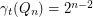.

Here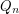denotes the-dimensional hypercube, i.e. the graph with vertex set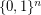and two vertices adjacent if they differ in exactly one coordinate. A total dominating set of a graphis a setof vertices ofsuch that every vertex has at least one neighbor in". The total domination numberofis the cardinality of a minimum total dominating set. A total dominator coloring of a graph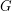, briefly TDC, is a proper coloring ofin which each vertex of the graph is adjacent to every vertex of some color class. The total dominator chromatic number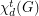ofis the minimum number of color classes in a TDC in(see [Kaz1]).

The following theorems are proved in [Kaz2].

Theorem   For any integer,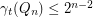.
Theorem   1. If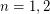, then.
2. If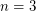, then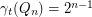.
3. If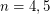, then.
Theorem   For any integer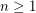,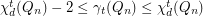.

## Bibliography

[Kaz1] Adel P. Kazemi, Total dominator chromatic number of a graph, http://arxiv.org/abs/1307.7486.

[Kaz2] Adel P. Kazemi, Total Dominator Coloring in Product Graphs, Utilitas Mathematica (2013), Accepted.

* indicates original appearance(s) of problem.

### The conjecture is false

The conjecture is false and it can be seen that this is so by using a computer program. For example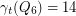which is not a power of two.

Alternatively we can argue as follows. For any graphwe clearly have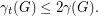Now the bound by Alon and Spencer statesIn particular this implies that for any constant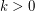and large enoughwe have